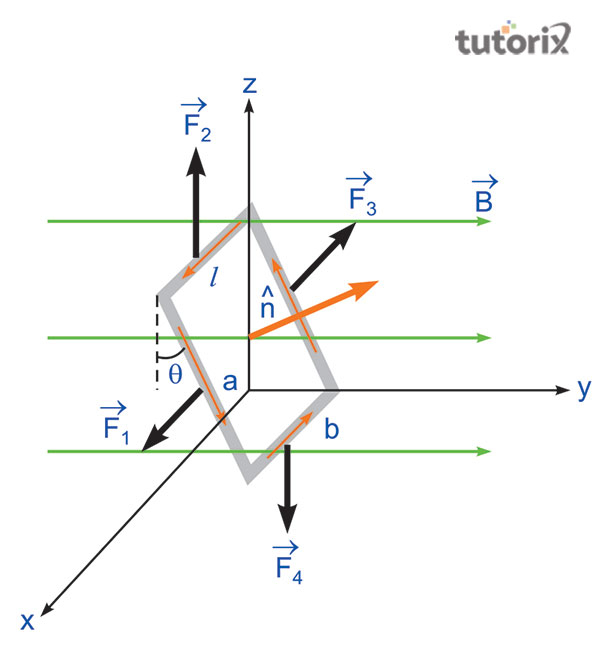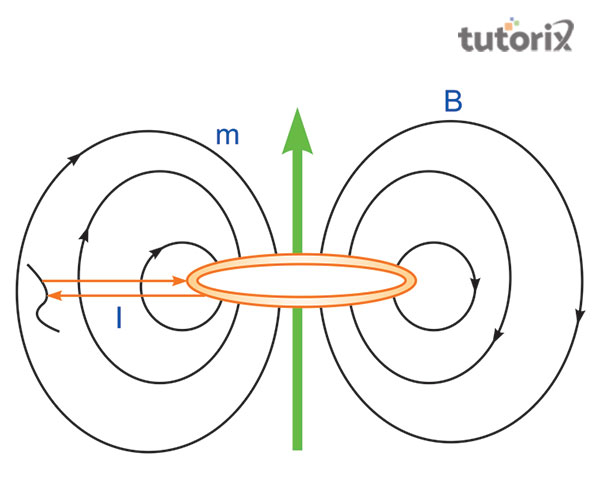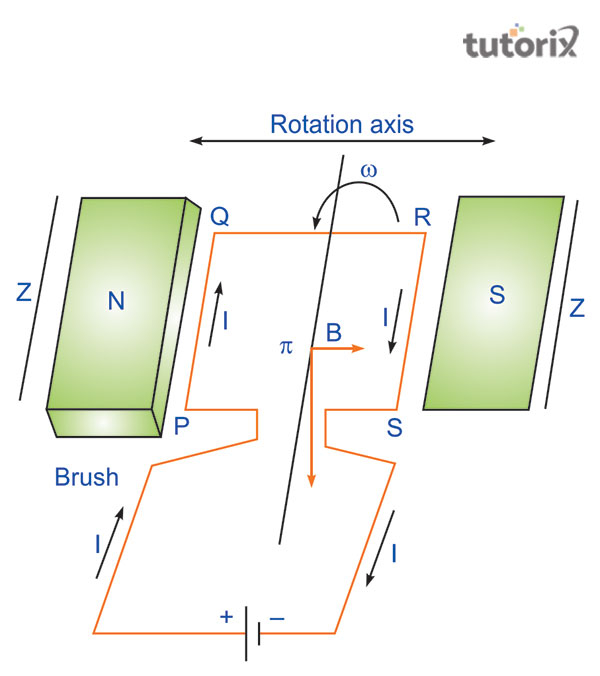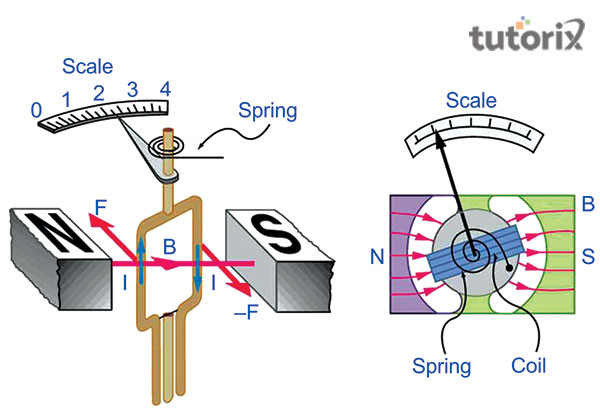# Torque Current Loop

Torque is demonstrated as a twisting force that has the tendency to create rotation. The rotation axis is illustrated as the point where the material moves. Torque on a current loop is basically demonstrated through a shape of rectangle kept in a uniform field of magnet that faces a torque. Torque is extremely important as it assists in determining whether the force applied may result in a clockwise or counter clockwise motion.

## What is torque?

Torque is defined as the rotational force to move the object concerning a pivot, axis and fulcrum. In the current loop of torque, electrical energy is altered to the mechanical work in the procedures (Alghamdi et al. 2019). Torque is reliable on multiple things such as the force magnitude, length between point of the axis of rotation and application of force and orientation of force concerning the relegation from the application of force point to the axis.Figure 1: Rectangular current loop in a uniform magnetic field

Torque's magnitude is calculated with the assistance of the formula, T = Newton-metre (Nm). N is discussed as a force unit and m is the metre, the distance unit. The torque (T) on a current-loop of a different structure in a uniform magnetic field is T = NIAB sin θ (Stiehl et al. 2019).

According to this, N denotes number of turns, I represents current, A is loop area, and B is the strength of magnetic field and θ denotes the angle between perpendiculars to the magnetic field and loop.

## What is magnetic dipole moment?

A magnetic dipole is defined as a magnetic north pole and south pole separated by a little distance. The Magnetic dipole moment possesses current time’s area dimensions or the energy dissected by the density of magnetic flux (Cao & Thurlbeck, 2019). Dipole moment’s unit is ampere square metre.Figure 2: Dipole moment of a magnet

The power of a tiny magnet or the dipole moment of magnet is provided by m = NIA, which is a vector quantity. The direction of the dipole moment of the magnet is obtained by the rule of right-hand thumb.

## Torque on current loop- magnetic moment

A dipole of the magnet is referred to as the confinement of a different closed loop of electric current or pole pairs as source dimensions are minimised to zero (Kurmann & Duarte, 2019). It is decreased to zero while letting the magnetic moment be completely constant.

## Case 1: Plane of a loop along a magnetic field

Consider the case when a loop of a rectangle is kept like the B (Uniform field of a magnet) is a loop plane. No pressure is exerted by the field on the loop arms and exhibited the force on which it is focused into the loop plane. The magnitude is demonstrated as F1 = IzB.

In the same way, it exhibits a force F2 on that lineF2 and RS which is showed out of the paper plane. Mathematically, F2 = IzB = F1. Hence, loop's net force is zero. Torque of the loop tends to revolve in the direction of anticlockwise.

T = F1 (y/2) = F2 (y/2)

= IzB (y/2) = IzB (y/2)

= I (y x z) B

= IAB……… (1)Figure 3: Magnetic moment of torque on the current loop

## Case 2: Plane of the loop not along magnetic field

Consider the case when the loop plane is not over the field of the magnet but creates an angle with it. Taking the angle between the normal and the field to the coil is represented as θ. The arm RS and PQ are F1 and F2. Hence, they are also opposite and equal in the magnitude,

F1 = F2 = IzB.

It is not represented as collinear as its consequences in a couple. The impact of torque is minimal as compared to the previous case when the loop plane was along the field of a magnet. The torque magnitude on the loop is

T= F1 (y/2) sinθ+ F2 (y/2) sinθ

= I (y×z) B sinθ

= IABsinθ …….(2)

So, combining both the calculations is represented as a vector product of the moment of the coil magnet and the phase of the magnet. Magnetic moment of the recent loop as m = IA.

## Torque on a Current Loop: Motors and Metres

Motors in physics are regarded as the general application of the force of a magnet on the current-carrying wires. Motors possess wire loops in the field of magnet (Kholmetskii, Missevitch & Yarman, 2020). The field of the magnet exhibits torque on the loops which rotate as a shaft.Figure 4: Motors and metres

Metres like that in the analogue fuel gauges on a car are defined as the torque application of a magnet on the current-carrying loop. The metre's construction is almost similar to that of the motors. The torque is adequately large to be beneficial to the motor (Kawaharazuka et al. 2019). The torque of the metre is directly comparable to the loop carried by the current and not θ. So, in metres, the deflection of the needle is comparable to that of current.

## Conclusion

Torque also assists in predicting the form of angular acceleration from which a certain material is being revolved. Torque is calculated in distinct units since it can be large or small and can feasibly be converted into any type of unit with the assistance of an online torque converter. The dipole moment of a magnet is considered to be an essential mechanism for the relaxation of the magnet between two protons or between an electron and proton in NMR. The context of torque and the magnetic moment has been observed through the equation.

## FAQs

Q1. What is the torque formula for current loop?

The formula of Torque on a loop is demonstrated utilising T = NIAB sin θ. The largest torque happens when θ is equal to 90° and Sin θ is equal to 1.

Q2. What is the net torque on a loop of the current?

Zero is the net torque performing on the loop. Hence, it stops revolving and the loop must be in the equilibrium state.

Q3. What is m in torque?

The Newton-metre is demonstrated as the torque unit in the SI system. I Nm is equal to torque consequence from a one-Newton force that is put on perpendicular to the moment end.

Q4. Why does torque maximise with distance?

Torque maximises with the distance as there is a wide distance to cover at a similar angle at the larger length from the axis where the arc is wider. It can be put over a wide distance if work requires the same.

Updated on: 18-Aug-2023

329 Views